Definitions of Square Dance Calls and Concepts

Index -->  Plus  |  A1  |  A2  |  C1  |  C2  |  C3A  |  C3B  |  C4  |  NOL  |
Definitions (Text Only) -->  Plus  |  A1  |  A2  |  C1  |  C2  |  C3A  |  C3B  |  C4  |  NOL  |
 Find call:

 \$B8@8l(Bview (admin)

\$BJ?9T\$J(B Line | Wave \$B\$+\$i(B.

Magic Line | Wave \$B\$O(B 1 \$B\$D\$N(B Line \$B\$N(B Ends \$B\$,B>\$N(B Line \$B\$N(B Centers \$B\$HF0\$/(B Distorted (\$B\$f\$,\$s\$@(B) Line | Wave \$B\$G\$9(B.

Magic Line | Wave: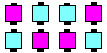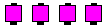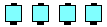\$B\$3\$N?MC#\$GF0\$-\$^\$9(B... \$B\$3\$3\$K\$\$\$k\$H;W\$\$(B... \$B\$^\$?\$O\$3\$3(B.

\$B%R%s%H(B: \$B%3!<%i!<\$,(B Magic Line | Wave \$B\$H8@\$C\$?\$i(B, \$BMagic Line | Wave \$B\$G\$N%Q!<%H%J!<\$H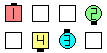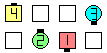Magic WaveSwitch The Wave\$B\$NA0(B \$B8e(B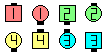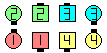Magic LineHere Comes The Judge\$B\$NA0(B \$B8e(B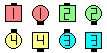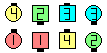Magic WaveIn Roll Circulate\$B\$NA0(B \$B8e(B

\$BCmMagic Line In Roll Circulate, Out Roll Circulate, Here Comes The Judge, Sock It To Me \$B\$N\$h\$&\$J%3!<%k\$G\$O(B, Circulate \$B\$r\$9\$k?M\$O(B, \$Be\$2\$F(B 'here' \$B\$^\$?\$O(B 'me', \$BF|K\\$G\$O(B '\$B\$O\$\$(B' \$B\$J\$I\$N@<\$r=P\$7\$F<+J,<+?H\$r3NG'\$9\$Y\$-\$G\$9(B. Circulate \$B\$r\$9\$k?M\$O(B, End \$B\$N0LCV\$+\$i\$b\$&(B 1 \$B\$D\$N(B End \$B\$N0LCV\$XF0\$/\$N\$G(B, \$BIaDL\$K(B Circulate \$B\$r\$7\$^\$9(B.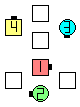Magic WaveSwitch To A Diamond\$B\$NA0(B \$B8e(B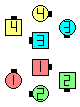Magic WaveSwitch To A Diamond\$B\$NA0(B \$B8e(B

Magic Wave \$B\$K\$\$\$??M\$I\$&\$7\$,(B Magic Diamond \$B\$K\$\$\$k\$3\$H\$r3NG'\$7\$F\$/\$@\$5\$\$(B.

\$BDL\$jJ}\$NNc(B:

• Magic Wave In Roll Circulate:
\$BJ?9T\$J(B Wave \$B\$+\$i(B. \$BCf\$r8~\$\$\$?(B Ends \$B\$H30\$r8~\$\$\$?(B Centers \$B\$O(B Circulate \$B\$r\$7(B, \$BB>\$N?M\$O(B Split Circulate twice \$B\$r\$7\$^\$9(B. \$BJ?9T\$J(B Wave \$B\$G=*\$o\$j\$^\$9(B. \$B\$3\$NDL\$jJ}\$O(B Wave \$B\$+\$i\$N\$H\$-\$@\$1\$G\$9(B.
• Magic Line Wheel & Spread:
\$BJ?9T\$J(B Inverted Line \$B\$+\$i(B. \$B8~\$\$9g\$C\$F\$\$\$k?M\$,(B Pass Thru \$B\$r\$7\$F(B; \$BA40w\$G(B Wheel & Spread \$B\$r\$7\$F(B; \$B%*%j%8%J%k\$N(B Magic Line \$B\$K\$\$\$J\$\$?M\$,(B Pass Thru \$B\$r\$7\$F(B, \$B%*%j%8%J%k\$N(B Magic Line \$B\$N0LCV\$G=*\$o\$j\$^\$9(B. \$BJ?9T\$J(B Inverted Line \$B\$G=*\$o\$j\$^\$9(B.
• Magic Wave Spin Chain Thru:
\$BJ?9T\$J(B Wave \$B\$+\$i(B. Magic Arm Turn 1/2 (Split Circulate Twice \$B\$N\$h\$&\$KF0\$-\$^\$9(B) \$B\$r\$7(B; Centers Cast Off 3/4; Very Centers Trade; Centers Cast Off 3/4 \$B\$r\$7\$^\$9(B. \$BJ?9T\$J(B Wave \$B\$G=*\$o\$j\$^\$9(B.

\$BCm

Magic Lines Of 3: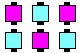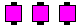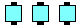\$B\$3\$N?MC#\$GF0\$-\$^\$9(B... \$B\$3\$3\$K\$\$\$k\$H;W\$\$(B... \$B\$^\$?\$O\$3\$3(B.

\$BMagic Diamond CONCEPT [C4] \$B\$H(B Trapezoid CONCEPT [C3B].Comments? Questions? Suggestions?

https://www.ceder.net/def/magicline.php?level=master&language=japan
24-May-2022 06:17:47